Courses

# Past Year Questions: Traffic Engineering GATE Notes | EduRev

## GATE : Past Year Questions: Traffic Engineering GATE Notes | EduRev

The document Past Year Questions: Traffic Engineering GATE Notes | EduRev is a part of the GATE Course Transportation Engineering.
All you need of GATE at this link: GATE

Question 1. The speed-density relationship of a highway is given as
u = 100 - 0.5k
where, u = speed in km per hour, k = density in vehicles per km. The maximum flow (in vehicles per hour, round off to the nearest integer) is _____     [2019 : 2 Marks, Set-II]
Solution: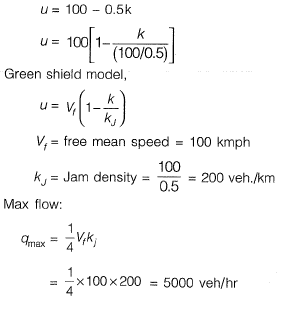Question 2. The uniform arrival and uniform service rates observed on an approach road to a signalized intersection are 20 and 50 vehicles/minute, respectively. For this signal, the red time is 30 s, the effective green time is 30 s, and the cycle length is 60 s. Assuming that initially there are no vehicles in the queue, the average delay per vehicle using the approach road during a cycle length (in s, round off to 2 decimal places) is______ .    [2019 : 2 Marks, Set-II]
Solution:
Arrival rate = 20 veh/min
Service rate = 50 veh/min
R= 30 sec, G1 = 30 sec, Cycle length = 60sec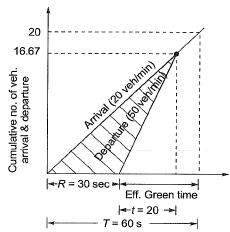Time corresponding to which no. of arrival becomes same as no. of departure.
=> 20 x (R + t) =50t
20 x (30 + t) =50t
600 + 20t =50t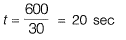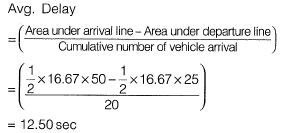Question 3. A vehicle is moving on a road of grade +4% at a speed of 20 m/s. Consider the coefficient of rolling friction as 0.46 and acceleration due to gravity as 10 m/s2. On applying brakes to reach a speed of 10 m/s, the required braking distance (in m, round off to nearest integer) along the horizontal, is     [2019 : 1 Mark, Set-II]
Solution: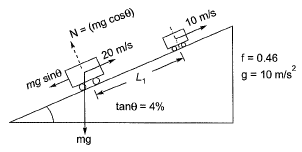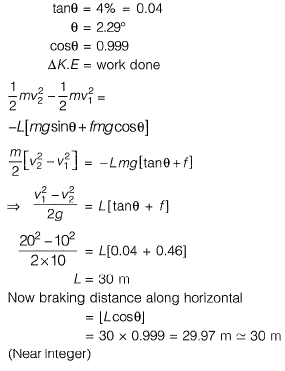Question 4.  The speed-density relationship in a mid-block section of a highway follows the Greenshield's model. If the free flow speed is vf and the jam density is kj, the maximum flow observed on this section is
(a)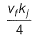(b)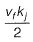(c)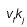(d)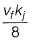(a)
Solution: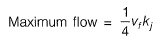Question 5. Traffic on a highway is moving at a rate of 360 vehicles per hour at a location. If the number of vehicles arriving on this highway follows Poisson distribution, the probability (round off to 2 decimal places) that the headway between successive vehicles lies between 6 and 10 seconds is_______.    [2019 : 2 Marks, Set-I]
Solution: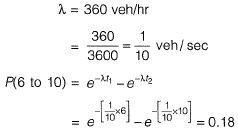Question 6.  Average free flow speed and the jam density observed on a road stretch are 60 km/h and 120 vehicles/km, respectively. For a linear speed- density relationship, the maximum flow on the road stretch (in vehicles/h) is_______ ,     [2019 : 2 Marks, Set-I]
Solution: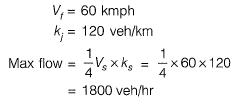Question 7. The maximum number of vehicles observed in any five minute period during the peak hour is 160. If the total flow in the peak hour is 1000 vehicles, the five minute peak hour factor (round off to 2 decimal places) is_______ .    [2019 : 1 Mark, Set-I]
Solution: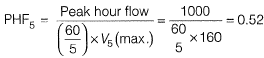Question 8. The space mean speed (kmph) and density (vehicles/km) of a traffic stream are linearly related. The free flow speed and jam density are 80 kmph and 100 vehicles/km respectively. The traffic flow (in vehicles/h, up to one decimal place) corresponding to a speed of 40 kmph is  _________    [2018 : 2 Marks, Set-II]
Solution:
Vf - Free mean speed = 50 kmph
kj = Jam density = 100 veh/km
As per linear model (green-shield)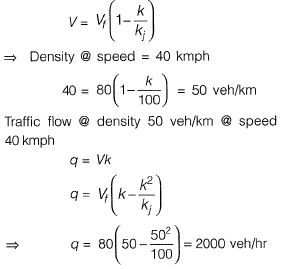Question 9. Peak Hour Factor (PHF) is used to represent the proportion of peak sub-hourly traffic flow within the peak hour. If 15-minute sub-hours are considered, the theoretically possible range of PHF will be     [2018 : 1 Mark, Set-II ]
(a) 0 to 1.0
(b) 0.25 to 0.75
(c) 0.25 to 1.0
(d) 0.5 to 1.0
Solution: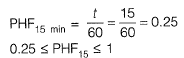Question 10. A well-designed signalized intersection is one in which the
(a) crossing conflicts are increased
(b) total delay is minimized
(c) cycle time is equal to the sum of red and green times in all phases
(d) cycle time is equal to the sum of red and yellow times in all phases     [2018 : 1 Mark, Set-I]

Question 11. The speed-density relationship for a road section is shown in the figure    [2018 : 1 Mark, Set-I]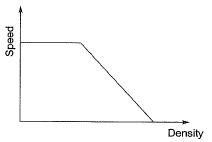The shape of the flow-density relationship is
(a) piecewise linear
(b) parabolic
(c) initially linear then parabolic
(d) initially parabolic then linear
Solution: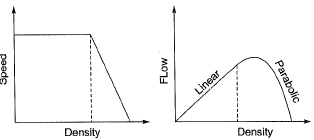q = vk
For initial values of k, v is constant
∴ q will very linearly with k
Later on, v varies linearly with k.
∴ q will vary parabolically with k.

Question 12. Two cars P and Q are moving in a racing track continuously for two hours. Assume that no other vehicles are using the track during this time. The expressions relating the distance travelled d (in km) and time t (in hours) for both the vehicles are given as
P : d=60t
Q : d = 60t2
Within the first one hour, the maximum space headway would be    [2017 : 2 Marks, Set-II]
(a) 15 km at 30 minutes
(b) 15 km at 15 minutes
(c) 30 km at 30 minutes
(d) 30 km at 15 minutes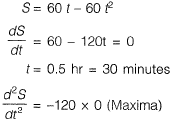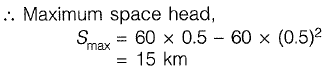Question 13. The safety within a roundabout and the efficiency of a roundabout can be increased, respectively by    [2017 : 2 Marks, Set-II]
(c)

Question 14. The queue length (in number of vehicles) versus time (in seconds) plot for an approach to a signalized intersection with the cycle length of 96 seconds is shown in the figure (not drawn to scale).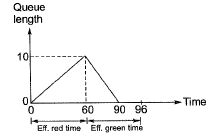At time, t = 0, the light has just turned red. The effective green time is 36 seconds, during which vehicles discharge at the saturation flow rate, s (in vph). Vehicles arrive at a uniform rate, v(in vph), throughout the cycle. Which one of the following statements is TRUE?    [2017 : 2 Marks, Set-I]
(a) v = 600 vph, and for this cycle, the average stopped delay per vehicle = 30 seconds.
(b) s = 1800 vph, and for this cycle, the average stopped delay per vehicle = 28.125 seconds.
(c) v = 600 vph, and for this cycle, the average stopped delay per vehicle = 45 seconds.
(d) s = 1200 vph, and for this cycle, the average stopped delay per vehicle = 28.125 seconds.
(b)
Solution: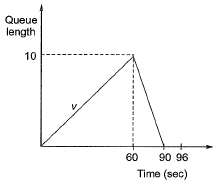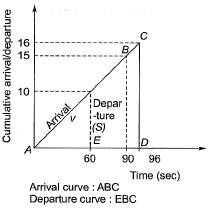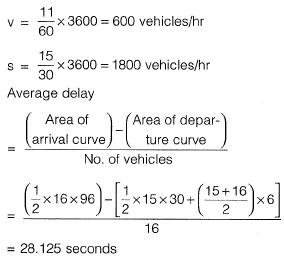Question 15. Vehicles arriving at an intersection from one of the approach roads follow the Poisson distribution. The mean rate of arrival is 900 vehicles per hour. If a gap is defined as the time difference between two successive vehicle arrivals (with vehicles assumed to be points), the probability (up to four decimal places) that the gap is greater than 8 seconds is __________.     [2017 : 1 Mark, Set-I]
Solution:

λ = 900 veh/hour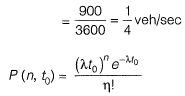Probability that time gap (time headway) betwen successive vehicles is greater than 8 seconds,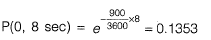Question 1. The critical flow ratios for a three-phase signal are found to be 0.30, 0.25, and 0.25. The total time lost in the cycle is 10 s. Pedestrian crossings at the junction are not significant. The respective Green times (expressed in seconds and rounded off to the nearest integer) for the three phases are    [2016 : 2 Marks, Set-II]
(a) 34, 28 and 28
(b) 40, 25, and 25
(c) 40, 30 and 30
(d) 50, 25, and 25
(a)
Solution: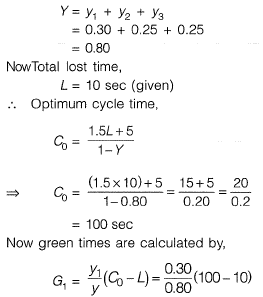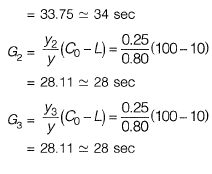Question 2.  If the total number of commercial vehicles per day ranges from 3000 to 6000, the minimum percentage of commercial traffic to be surveyed for axle load is     [2016 : 1 Mark, Set-II]
(a) 15
(b) 20
(c) 25
(d) 30
(a)

Question 3. While traveling along and against the traffic stream, a moving observer measured the relative flows as 50 vehicles/hr and 200 vehicles/hr, respectively. The average speeds of the moving observer while traveling along and against the stream are 20 km/hr and 30 km/hr, respectively. The density of the traffic stream (expressed in vehicles/km) is________.      [2016 : 2 Marks, Set-I]
Solution:

Method-I
Let us assume,
q = traffic flow
k = traffic density
V = velocity of test vehicle along the stream
V - velocity of test vehicle against the stream
=> q - KVw =50
=> q - k x 20 = 50 ...(i)
q + kVa = 200
=> q + k x 30 = 200 ...(ii)
Solving equation (i) and (ii), we get
k = 3 Veh/km
Method-ll
Consider a ‘L’ km long stretch,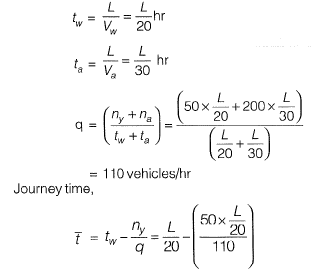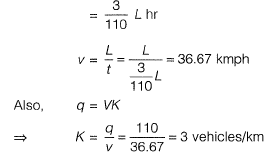Question 4. Inaone-lane one-way homogeneous traffic stream, the observed average headway is 3.0s. The flow (expression vehicles/hr) in this traffic stream is __________    [2016 : 1 Mark, Set-I]
Solution:
= 3.0 sec
Flow of traffic stream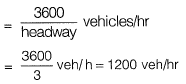Quesion 5. The relation between speed u(in km/h) and density k (number of vehicles/km) for a traffic stream on a road is u = 70 - 0.7k. The capacity on this road is ________ vph (vehicles/hour).     [2015 : 2 Marks, Set-II]
Solution:
Traffic volume,
q= uk
q= (70-0.7 k)k
= 70k-0.7 k2
Maximum possible volume is capacity of road
For maximum volume,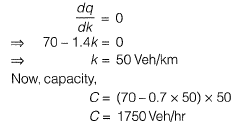Question 6. The following statements are made related to the lengths of turning lanes at signalised intersections
(i) 1.5 times the average number of vehicles (by vehicle type) that would store in turning lane per cycle during the peak hour.
(ii) 2 times the average number of vehicles (by vehicle type) that would store in turning lane per cycle during the peak hour.
(iii) Average number of vehicles (by vehicle type) that would store in the adjacent through lane per cycle during the peak hour.
(iv) Average number number of vehicles (by vehicle type) that would store in all lanes per cycle during the peak hour.
As per the IRC recommendations, the correct choice for design length of storage lanes is    [2015 :1 Mark, Set-Il]
(a) Maximum of (ii) and (iii)
(b) Maximum of (i) and (iii)
(c) Average of (i) and (iii)
(d) Only (iv)

Solution: Design length is the average of (i) and (iii) as per Indian Roads Congress (IRC) recommendations for storage lanes.

Question 7.  Which of the following statements CANNOT be used to describe free flow speed (uf) of a traffic stream?    [2015 : 1 Mark, Set-I]
(a) uf is the speed when flow is negligible
(b) uf is the speed when density is negligible
(c) uf is affected by geometry and surface conditions of the road
(d) uf is the speed at which flow is maximum and density is optimum

Solution: Free flow speed (Vf) is that speed at which flow and density is negligible.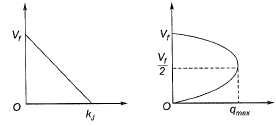Question 8.  A pre-timed four phase signal has critical lane flow rate for the first three phases as 200, 187 and 210 veh/hr with saturation flow rate of 1800 veh/hr/lane for all phases. The lost time is given as 4 seconds for each phase. If the cycle length is 60 seconds, the effective green time (in seconds) of the fourth phase i s ________ .     [2014 : 2 Marks, Set-II]
Solution:
Flow rates for the first three phase are given as
q1 = 200 veh/hr
q2 = 187 veh/hr
and q3 = 210 veh/hr
Saturation flow rate is 1800 veh/hr/lane
Lost time,
L = 4 x 4 = 16 sec
Length of the cycle,
C0 = 60 sec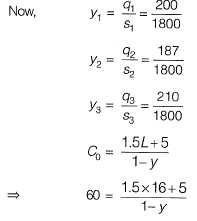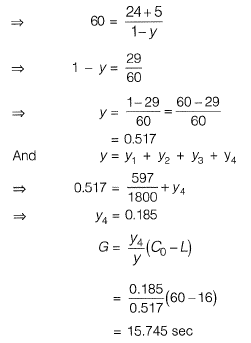Question 9. On a section of a highway the speed- density relationship is linear and is given by v = [80 - (2/3) k]; where v is in km/h and k is in veh/km. The capacity (in veh/h) of this section of the highway would be    [2014 : 2 Marks, Set-II]
(a) 1200
(b) 2400
(c) 4800
(d) 9600

Solution:
Method-I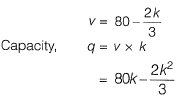Method-II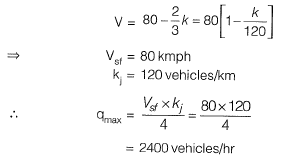Question 10. A student riding a bicycle on a 5 km one-way street takes 40 minutes to reach home. The student stopped for 15 minutes during this ride. 60 vehicles overtook the student (assume the number of vehicles overtaken by the student is zero) during the ride and 45 vehicles while the student stopped. The speed of vehicle stream on that road (in km/hr) is     [2014 : 2 Marks, Set-II]
(a) 7.5
(b) 12
(c) 40
(d) 60
Solution:

Method-I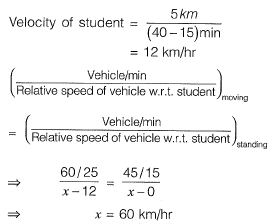Method-I
45 vehicles overtook the student when he stopped for 15 minutes.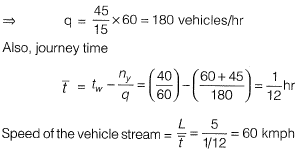Offer running on EduRev: Apply code STAYHOME200 to get INR 200 off on our premium plan EduRev Infinity!

## Transportation Engineering

23 videos|66 docs|39 tests

,

,

,

,

,

,

,

,

,

,

,

,

,

,

,

,

,

,

,

,

,

;